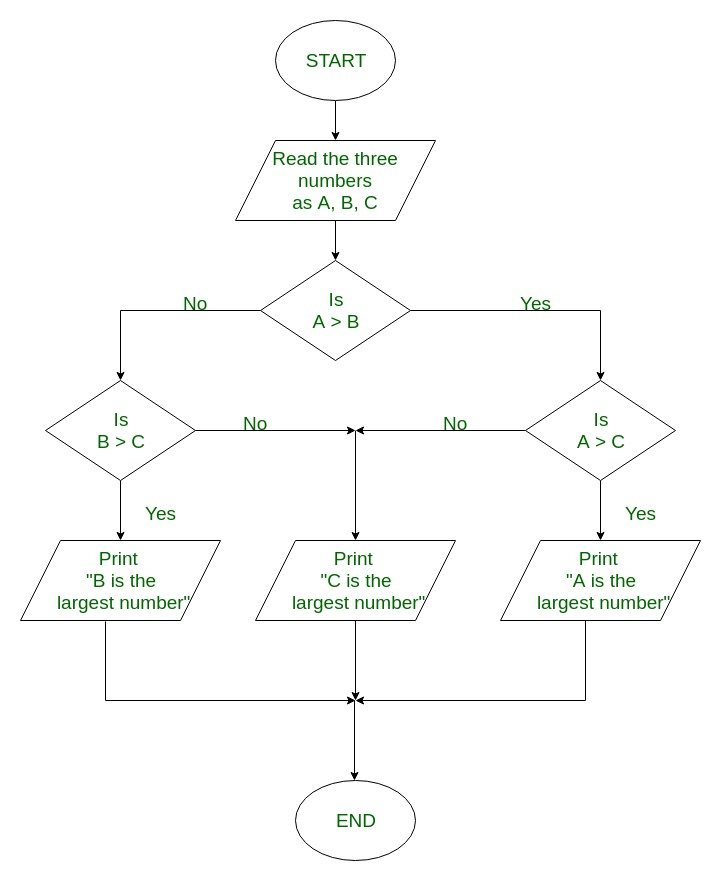Open in App
Not now

# Java Program to Find the Largest of three Numbers

• Difficulty Level : Easy
• Last Updated : 13 Sep, 2022

Problem Statement: Given three numbers x, y, and z of which aim is to get the largest among these three numbers.Example:

```Input: x = 7, y = 20, z = 56
Output: 56                    // value stored in variable z```

Flowchart For Largest of 3 numbers:Algorithm to find the largest of three numbers:

```1. Start
2. Read the three numbers to be compared, as A, B and C
3. Check if A is greater than B.

3.1 If true, then check if A is greater than C
If true, print 'A' as the greatest number
If false, print 'C' as the greatest number

3.2 If false, then check if B is greater than C
If true, print 'B' as the greatest number
If false, print 'C' as the greatest number
4. End```

Approaches:

• Using Ternary operator
• Using if-else

### Approach 1:Using Ternary operator

The syntax for the conditional operator:

`ans = (conditional expression) ? execute if true : execute if false`
• If the condition is true then execute the statement before the colon
• If the condition is false then execute a statement after colon so
`largest = z > (x>y ? x:y) ? z:((x>y) ? x:y); `

Illustration:

```x = 5, y= 10, z = 3

largest  = 3>(5>10 ? 5:10) ? 3: ((5>10) ? 5:10);
largest  = 3>10 ? 3 : 10
largest  = 10```

## Java

 `// Java Program to Find the Biggest of 3 Numbers` `// Importing generic Classes/Files``import` `java.io.*;` `class` `GFG {``  ` `    ``// Function to find the biggest of three numbers``    ``static` `int` `biggestOfThree(``int` `x, ``int` `y, ``int` `z)``    ``{` `        ``return` `z > (x > y ? x : y) ? z : ((x > y) ? x : y);``    ``}` `    ``// Main driver function``    ``public` `static` `void` `main(String[] args)``    ``{` `        ``// Declaring variables for 3 numbers``        ``int` `a, b, c;` `        ``// Variable holding the largest number``        ``int` `largest;``        ``a = ``5``;``        ``b = ``10``;``        ``c = ``3``;``        ``// Calling the above function in main``        ``largest = biggestOfThree(a, b, c);` `        ``// Printing the largest number``        ``System.out.println(largest``                           ``+ ``" is the largest number."``);``    ``}``}`

Output

`10 is the largest number.`

### Approach 2: Using the if-else statements

In this method, if-else statements will compare and check for the largest number by comparing numbers. ‘If’ will check whether ‘x’ is greater than ‘y’ and ‘z’ or not. ‘else if’ will check whether ‘y’ is greater than ‘x’ and ‘z’ or not. And if both the conditions are false then ‘z’ will be the largest number.

## Java

 `// Java Program to Find the Biggest of 3 Numbers` `// Importing generic Classes/Files``import` `java.io.*;` `class` `GFG {` `    ``// Function to find the biggest of three numbers``    ``static` `int` `biggestOfThree(``int` `x, ``int` `y, ``int` `z)``    ``{` `        ``// Comparing all 3 numbers``        ``if` `(x >= y && x >= z)` `            ``// Returning 1st number if largest``            ``return` `x;` `        ``// Comparing 2nd no with 1st and 3rd no``        ``else` `if` `(y >= x && y >= z)` `            ``// Return z if the above conditions are false``            ``return` `y;` `        ``else` `            ``// Returning 3rd no, Its sure it is greatest``            ``return` `z;``    ``}` `    ``// Main driver function``    ``public` `static` `void` `main(String[] args)``    ``{``        ``int` `a, b, c, largest;` `        ``// Considering random integers three numbers``        ``a = ``5``;``        ``b = ``10``;``        ``c = ``3``;``        ``// Calling the function in main() body``        ``largest = biggestOfThree(a, b, c);` `        ``// Printing the largest number``        ``System.out.println(largest``                           ``+ ``" is the largest number."``);``    ``}``}`

Output

`10 is the largest number.`

## Java

 `// Java Program to Find the Largest of three Numbers``// Using Collections.max() method` `import` `java.lang.*;``import` `java.util.*;` `class` `GFG {` `    ``public` `static` `void` `main(String[] args)``    ``{``        ``int` `a, b, c;``        ``// Considering random integers three numbers``        ``a = ``5``;``        ``b = ``10``;``        ``c = ``3``;``        ``ArrayList x = ``new` `ArrayList<>();``        ``x.add(a);``        ``x.add(b);``        ``x.add(c);` `        ``// Printing the largest number``        ``System.out.println(Collections.max(x)``                           ``+ ``" is the largest number."``);``    ``}``}`

Output

`10 is the largest number.`

My Personal Notes arrow_drop_up